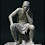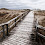## 20140516

### 哲學的啟發 --- 兩個實例

1. 烏鴉悖論（the paradox of the ravens

(a)  所有烏鴉都是黑色的。

(b)  所有非黑色的東西都不是烏鴉。

2.  二階意志（second-order volitions

Harry Frankfurt 的著名論文我已讀過無數次，因為選了它作形上學一科的教材，每次教時我都會重讀一遍。這篇論文內容豐富、立論獨特、例子有趣、文筆清通，任何對「自由意志和道德責任的關係」有興趣的人都應該一讀。雖然這篇論文不算艱深，而我第一次讀它時已是個研究生，但仍有不少地方是多年後重讀多次才理解得透徹；不過，其中「二階意志」這個重要概念，是初讀時就對我大有啟發的。

Frankfurt 先劃分了一階意欲（first-order desires）和二階意欲（second-order desires），以下是兩個簡單的例子：

(i)   我想戒酒。

(ii)  我希望自己有意欲戒酒。

(i) (ii) 都表達了我的意欲，而 (ii) 表達的意欲是二階的，因為這個意欲的對象是 (i) 表達的那個意欲 --- 以意欲為對象的意欲，就是二階意欲。

「二階意志」不是一個複雜的概念，當年我明白了之後，立刻視之為一個方便反思的工具：問一問自己有沒有二階意志，如果有，是哪些 --- 你會對自己有更深的了解；假如答案是「沒有」，便要思考該怎樣做 --- 如果不想繼續做 wanton，因而終於確定了一些二階意志，你的人生便可能有自主的方向。

#### 40 則留言:

1.烏鴉悖論 好有意思,
仔細推敲覺得都對, 卻心理怪怪的.

把黑色換成烏鴉色,
非烏鴉色的蘋果, 非烏鴉色的寶石...
支持 a 的說法就比較容易說服我...
(後來去找貝氏定理...果然有意思)

二階意志也是個有趣的想法,
不過當下我就落入三階意志的笨蛋想法...

1.落入三階意志 甚至四階也沒有問題
雖然在logic上你可以建立無限階既意志 但係心理上你永遠有限制的

2.觀察到白色的天鵝、紅色的蘋果、綠色的寶石等，又怎能支持「所有烏鴉都是黑色的」呢？//

因為(非黑的色的東西)B 都不是烏鴉的證據,增加了(所有烏鴉都是黑色)A 的可信性.
邏輯上想,的確是增加了. 既然同意B,就應該同意A 丫,我是這樣思考的.

yui

1.問題是，這樣理解的話，理論上便可以從未見過黑色的烏鴉，卻有大量的「證據」支持「所有烏鴉都是黑色的」。

3."烏鴉悖論" is misleading because the two hypotheses
(a) 所有烏鴉都是黑色的。
(b) 所有非黑色的東西都不是烏鴉。
actually are NOT logically equivalent. This can be shown when one negates the hypotheses:

(a) 所有烏鴉都是黑色的
NOT(a) 沒有烏鴉不是黑色的

(b) 所有非黑色的東西都不是烏鴉
NOT(b) 沒有"非黑色的東西"是烏鴉

But when doing a negation of NOT(b): i.e. NOT(NOT(b))
there are two possibilities:
有些黑色的東西是烏鴉
and
所有黑色的東西都是烏鴉

Thus the claim about (a) and (b) are logically equivalent breaks down.

As the two hypotheses are not logically equivalent, the "烏鴉悖論" doesn't exist.

1.CORRECTION:

"Thus the claim about (a) and (b) are logically equivalent breaks down."

should be

"Thus the claim about (a) and (b) **being** logically equivalent breaks down."

2.(b) is the contraposition of (a). Here's another way of explaining why they are logically equivalent:

(a) All ravens are black.
(b) Everything that isn’t black, isn’t a raven.

(a) says that everything of a particular kind has a certain property. (b) says that everything that lacks that property isn’t of that kind.

Please keep this in mind: the paradox of the ravens has been discussed by philosophers for decades. If (a) and (b) were not logically equivalent, someone would have pointed that out long time ago.

If you insist that you are right, I would advise you to write a paper showing that (a) and (b) are not logically equivalent and that there is no paradox of the ravens, and submit it to a journal of logic or journal of philosophy.

3.If (a) is logically equivalent to (b), then (a) has to be logically equivalent to NOT(NOT(b))), which is practically (b). My above post showed that NOT(NOT(b))) isn't logically equivalent to (a). Therefore there is no paradox of ravens.

4.//(b) 所有非黑色的東西都不是烏鴉
NOT(b) 沒有"非黑色的東西"是烏鴉//

- Are you saying "沒有非黑色的東西是烏鴉" is the negation of "所有非黑色的東西都不是烏鴉"?

4.(a) 所有烏鴉都是黑色的
NOT(a) 有些烏鴉不是黑色的

(b) 所有非黑色的東西都不是烏鴉
NOT(b) 有些"非黑色的東西"是烏鴉

NOT(a) = NOT(b)

The negation of “All P are Q” is “Some P are not Q”, but not “No P is Q” (= All P are not Q).

1.Thank you, Joe. But it seems that the guy thinks the negation of "All P are Q" is "No P are not Q"!

//a) 所有烏鴉都是黑色的
NOT(a) 沒有烏鴉不是黑色的//

2."NOT(a)" was never used.

3.//a) 所有烏鴉都是黑色的
NOT(a) 沒有烏鴉不是黑色的//

"沒有烏鴉不是黑色的" 是 "所有烏鴉都是黑色的" 的obverse（換質命題），兩者等義，可互相轉換。

observe 不是你所謂的 NOT negation。

後來，

//But when doing a negation of NOT(b): i.e. NOT(NOT(b))
//there are two possibilities:
//有些黑色的東西是烏鴉
//and
//所有黑色的東西都是烏鴉

這裡所謂兩個possibilities，第一個可以是NOT negation，但它是"所有黑色的東西都不是烏鴉"的negation；第二個則又是前述的obverse。你拿obverse來魚目混珠冒充negation，製造假的possibility！一個直言命題的negation根本不存在歧義的問題。

這麼明顯的錯誤，這麼基本的道理都搞混，還能進一步談什麼？

4.The mistake the guy has made is different from the mistake Joe has pointed out, although the mistake Joe has pointed out is also a mistake that people commonly made.

The guy has not mistaken the negation of "All P are Q" as "All P are not Q"; rather has he mistaken the negation of "All P are Q" as "No P are not Q", which actually means the same with "All P are Q".
(the guy says: (a)= 所有烏鴉都是黑色的; negation of (a)= 沒有烏鴉不是黑色的)

5.You are right! I slipped up!

5.(a): Y is Z
(b): non-Z is not Y

Not(b): non-Z is Y
Not(Not(b)): Z is Y

Is (a) logically equivalent to Not(Not(b)) ?

1.logic ...only T , F, 2 status.

not(T) = F , not(F) = T , this is the definition of 'not' operand in logic.

so,
(a) : Y is Z (this is statement, you could say "Y is Z" is true or false),
(b) : not Z is not Y (this is statement too).

not(b) , in logic , it means:
if b is true, then not(b) is false.
if b is false, then not(b) is true.

not(not Z is not Y) does not means not Z is Y...

2.Use brain first; ignore alphabets.

3.you have a misconception in negation.

Let (a): "A => B".

NOT(a) should be "A and NOT(B)". Or informally "there exists some condition satisfying A but not B"

4.You assumed.

5.//You assumed.//

Do you mean everyone can have his own definition of NOT?
I am not arguing with you what is the best definition of NOT. But if you insist on your definition of NOT, then " (b) is logically equivalent to NOT(NOT(b)) " does not hold.

It is what you mention before:

// then (a) has to be logically equivalent to NOT(NOT(b))), which is practically (b) //

And look at what you also mention:

//// (a): Y is Z
(b): non-Z is not Y

Not(b): non-Z is Y
Not(Not(b)): Z is Y ////

Does your (b) actually mean the same thing as your NOT(NOT(b)) ?

For example:
If we know only "X is not clever --> X is not rich",
can we deduce from it "X is clever --> X is rich" ?

6.You assumed that it has to be a logic operator.

This "paradox" matter has been solved. Different people might use different approach to uncover its mystery. Probably the logic table is the easiest and simplest way to demonstrate why (a) and (b) are not logical equivalent (as in below).

Because the source of the problem is Logic, applying logic operations would lead an investigator to go around full circles. Thus one needs to apply a different set of operators, hoping that they would do some good.

If you apply the known logic operations as if doing mathematics, (a) and (b) will always yield the SAME answer, implying that they are logical equivalent, leading to confirm the paradox. But human intelligence tells that there must be a catch in the paradox. Finding the catch solves the mystery.

The terms "ravens" and "black" create distractions, thus one needs to overlook the alphabets but go back to pure Logic mode. i.e. treating them as "symbols" instead of the words "ravens" and "black".

By introducing "r" for "T(rue) maybe", "A -> B" can no longer be rewritten as "~A or B", and that is the catch, as it was the source of the paradox in the first place.

One may eat with a fork, a spoon, a pair of chopsticks, a knife, a stick, and even with our own hands. And the case is closed.

6.The paradox of ravens happens because of a problem in the truth table:

Let M be "p->q" (for easy text formatting):

p q M
T T T
T F F
F T T
F F T

Suppose instead of T(rue) & F(alse), we allow a third state r for "T(rue) maybe".
The new truth table would be:

p q M
T T T
T F F
F T r
F F r

A: x is a raven
B: x is black
(a): All ravens are black ===> A -> B ===> ~A or B
(b): all non-blacks are non-ravens ==> ~B -> ~A ===> ~(~B) or ~A ===> B or ~A

A B a b
T T T T
T F F F
F T T T
F F T T

But if we introduce "r" for "T(rue) maybe".

A B a b
T T T r
T F F F
F T r r
F F r T

Now is (a) and (b) really logical equivalent?

From (b), at most one may deduce "some B is A" or "All B is A". It may never be used to deduce "All A is B".

1.(b) may deduce (a) only if "r" ["T(rue) maybe"] isn't used. Thus the paradox is due to a fault in the logic table, instead of something happening in our human world.

7.I'd like to express my view as a mathematician/computer scientist.

Evidence for (a) is drawn from a pool of finite ravens. whereas evidence for (b) must be drawn from a pool of infinite number of non-raven objects. It is possible to observe every raven on Earth and conclude that (a) is true. But it is impossible to observe every non-raven objects. Therefore, non-black non-raven objects are not evidences (or negligible evident).

Sirius

1.In reality, both (a) and (b) have infinite number of objects.

- - - -

The paradox is about "why seeing (b) could deduce (a)". Through Logic & its logical operations as shown above, (b) could actually be rewritten to become (a).

2.In case you are not convinced:

Since "raven" and "black" are just "labels", what if they are changed to something like "star" and "heavy".

3.You are correct that "raven" and "black" can be changed to anything, say, P and Q. But the raven paradox only occurs when the pool of P is significantly smaller than the pool of non-P. For example,

(c) All male lions have mane.
(d) All lions without mane are not male.

Since the number of male and non-male (female) lions are roughly equal, we are able to naturally take evidence for (d) as also evidence for (c). And the raven paradox does not occur.

Sirius

4.Pure Logic doesn't care about "ravens", "lions", population and quantity. The latter are for Statistics.

Just pretend you are Spock of "Star Trek", will you?

5.As a computer scientist, I am trained in both logic and statistics. I am very well aware that pure logic doesn't care about population and quantities. But I have clearly stated that I am offering a different point of view using mathematics right at the beginning of my comment. Unless you have arguments to support you, please don't call my view wrong. You know you don't have to comment.

BTW, Spork would be able to use statistical methods as well.

Sirius

6.The paradox cannot be solved statistically because it is a side-effect of a fault in the Logic tool-set, rather than a problem caused by the population of ravens or lions.

8.//(a) 所有烏鴉都是黑色的。(b) 所有非黑色的東西都不是烏鴉。//

問題相信是因為用了「黑色」這個基本上只描述了一個特徵的詞（涵蓋面很寬），來代表了還有很多其它特徵的「烏鴉」（比黑色這個詞的涵蓋面少得多）；這就好比說用「黃色」來代表「中國人」那樣，於是考究「中國人」就變為了考究「黃色」的東西那樣未必都是與中國人有關。
可能這個假設是要有一個前者的涵蓋面不能夠大於後者才有意義，就像數學裡的分數那樣，要分母不為0才有意義一樣。

1.>> 就像數學裡的分數那樣，要分母不為0才有意義一樣。

Thus we have Calculus.

2.//You have misunderstood the paradox. //

那麼請問如果把「黑色」這個涵蓋面很寬的詞改為涵蓋面不大於「烏鴉」這個詞的涵蓋面，例如改為：

(a) 所有烏鴉都是這種DNA結構的。(b) 所有非這種DNA結構的東西都不是烏鴉。

3.4.或者應該是這樣說，把涵蓋面收窄的意思是要令(b)句不會使得例如像紅色的蘋果和綠色的寶石等等很多無關的東西能夠被納入考慮之列，例如試這樣說

(a) 所有烏鴉都是這種DNA的鳥。(b) 所有非這種DNA的鳥都不是烏鴉。

5.A: x is M
B: x is N
(a): A -> B
(b): ~B -> ~A

This is a paradox when M is not N.

6.//This is a paradox when M is not N. //

黑色, 鳥, DNA相信只是涵蓋了烏鴉，但都不是烏鴉，只是涵蓋範圍收窄。
那麼：
(a) 所有烏鴉都是這種DNA的鳥。(b) 所有非這種DNA的鳥都不是烏鴉。

7.Anything that you could put into this form will (most likely??) be a paradox:

A: x is M
B: x is N
(a): A -> B
(b): ~B -> ~A

The uncertainty is in case you put things like "God" or "infinite" into M and N, trying to test its limit.

9.「二階意志」可能是屬於對為什麼要做一件事有更深層次的理解；若去到「三四階意志」的話，恐怕就是屬於「信仰」的地步了？